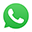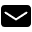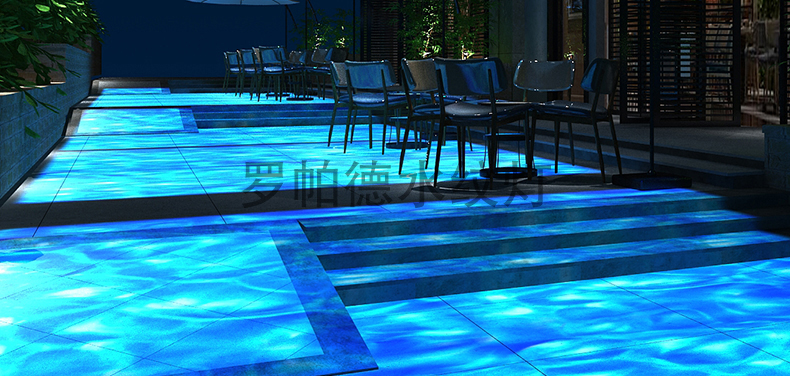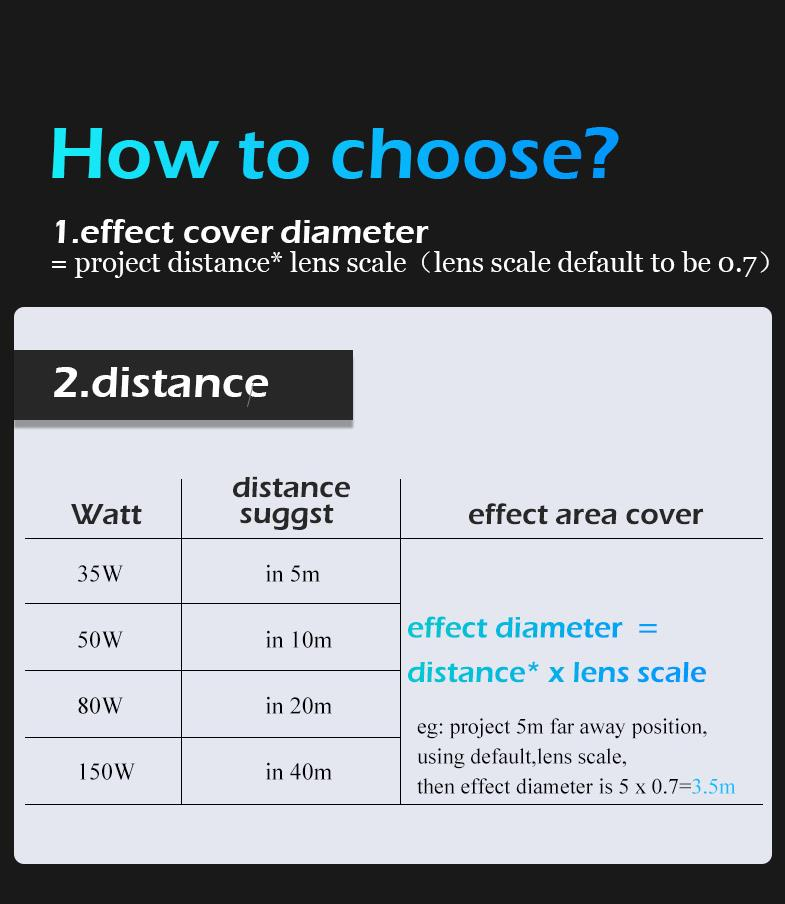+86 18126095160bella@noparde.com

Home > Blog >  What is the irradiated area of the water-waving projector?

### What is the irradiated area of the water-waving projector?

2021-12-14  352

The water-waving projector product projects the lamp sheet's water pattern onto the ground outside through strong light, forming a microwave sparkling water ripple. Using a water-waving projector is not enough to achieve the effect of brightening a certain venue. So at this time, we need to know the irradiation area of a water-waving projector. How many water-waving projectors do we need to complete the water-waving projector lighting project? The following is the irradiation area of the water-waving projector introduction.The main factor that affects the irradiation area of the water-waving projector is the projector's lens. We have 0.2 lens, 0.33 lens, 0.45 lens, 0.7 lens. We often recommend a 0.7 lens to the customers. Using 0.7 lenses to project is 0.7 meters, and the greater the distance, the larger the water waving pattern. But we need to suitable power water waving projector. How many power water-waving projectors do we need? You can contact the staff in Noparde projector.

All in all, the following is the calculating formula for the irradiated area of the water-waving projector: the size of the irradiated area =projection distance* lens ratio. For example, the projection distance is 5 meters, and choosing the projection lens is 0.7, so the water waving pattern is 5*0.7=3.5 meters.The above are affecting factors and calculating formula for the irradiated area of the water waving projector. Not only can the calculating formula applies to the water-waving projector, but also every projector in the Noparde projector. But, we should pay attention to the water-waving projector; it can be a coincident projection. Other pattern projectors also consider the projection environment; for example, the above formula cannot be used when projecting on a special-shaped building.